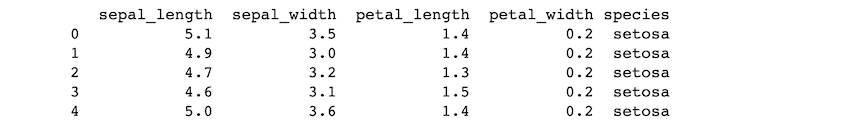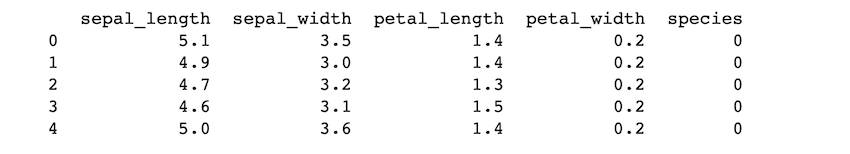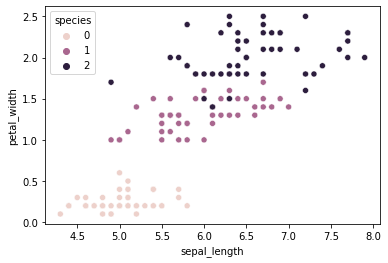# Create a Scatter Plot using Sepal length and Petal_width to Separate the Species Classes Using scikit-learn

In this article, we are going to see how to create Scatter Plot using Sepal length and Petal_width to Separate the Species classes using scikit-learn in Python.

The Iris Dataset contains 50 samples of three Iris species with four characteristics (length and width of sepals and petals). Iris setosa, Iris virginica, and Iris versicolor are the three species. These measurements were utilized to develop a linear discriminant model to classify the species. The dataset is frequently used in data mining, classification, clustering, and algorithm testing.

Now, let’s create a scatter plot using Sepal length and petal width to separate the species classes using scikit-learn.

## Import the data

First, let’s import the packages and load the “iris.csv” file. The .head() method returns the first five rows of the dataset. The columns in our dataset are ‘sepal_length’, ‘sepal_width’, ‘petal_length’, ‘petal_width’ and ‘species’.

## Python3

 `# importing packages ` `import` `pandas as pd ` `import` `matplotlib.pyplot as plt ` `from` `sklearn ``import` `preprocessing ` `import` `seaborn as sns ` ` `  `# loading data ` `iris ``=` `pd.read_csv(``"iris.csv"``) ` `print``(iris.head()) `

Output:## Label encoding the ‘species’ column of the dataset

sklearn.preprocessing.LabelEncoder() converts string labels to numerical labels. After encoding the ‘species’ column the dataset looks like this:

## Python3

 `le ``=` `preprocessing.LabelEncoder() ` ` `  `# Converting string labels of ` `# the 'species' column into numbers. ` `iris.species ``=` `le.fit_transform(iris.species) ` `print``(iris.head()) `

Output:## Creating a scatterplot

We use the matplotlib and seaborn libraries to create a scatterplot. sns.scatterplot() is used to create a scatterplot, as we need to visualize sepal length and petal width, on the x-axis we give ‘sepal_length’, and on the y-axis we give ‘petal_width’, hue parameter is for the color on the plot, we gave the column name ‘species’ for that parameter as we want to differentiate the data among the species and the column is already label encoded. The new labels are 0,1,2. In the legend, we can see that. The species are classified in the scatter plot according to the labels.

## Python3

 `# plotting a scatterplot using seaborn ` `sns.scatterplot(data``=``iris, x``=``'sepal_length'``, ` `                ``y``=``'petal_width'``, hue``=``'species'``) ` `plt.plot() `

Output:Whether you're preparing for your first job interview or aiming to upskill in this ever-evolving tech landscape, GeeksforGeeks Courses are your key to success. We provide top-quality content at affordable prices, all geared towards accelerating your growth in a time-bound manner. Join the millions we've already empowered, and we're here to do the same for you. Don't miss out - check it out now!

Previous
Next